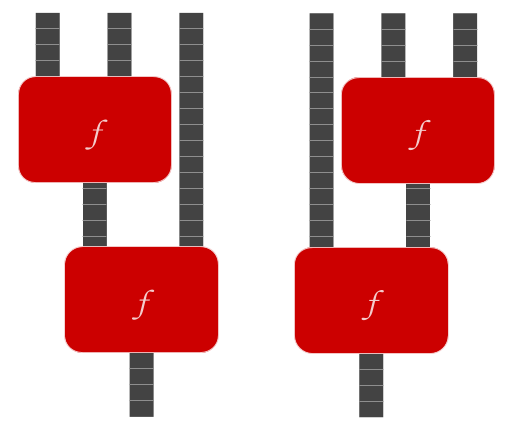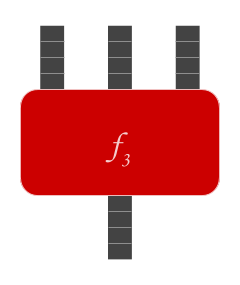# Associative operation

An associative operation $$\bullet : X \times X \to X$$ is a binary operation such that for all $$x, y, z$$ in $$X$$, $$x \bullet (y \bullet z) = (x \bullet y) \bullet z$$. For example, $$+$$ is an associative function, because $$(x + y) + z = x + (y + z)$$ for all values of $$x, y,$$ and $$z$$. When an associative function is used to combine many elements in a row, parenthesis can be dropped, because the order of application is irrelevant.

Imagine that you’re trying to use $$f$$ to combine 3 elements $$x, y,$$ and $$z$$ into one element, via two applications of $$f$$. $$f$$ is associative if $$f(f(x, y), z) = f(x, f(y, z)),$$ i.e., if the result is the same regardless of whether you apply $$f$$ to $$x$$ and $$y$$ first (and then apply that result to $$z$$), or whether you apply $$f$$ to $$y$$ and $$z$$ first (and then apply $$x$$ to that result).

Visualizing $$f$$ as a physical mechanism, there are two different ways to hook up two copies of $$f$$ together to create a function $$f_3 : X \times X \times X \to X,$$ which takes three inputs and produces one output:An associative function $$f$$ is one where the result is the same no matter which way the functions are hooked up, which means that the result of using $$f$$ twice to turn three inputs into one output yields the same output regardless of the order in which we combine adjacent inputs.By similar argument, an associative operator $$f$$ also gives rise (unambiguously) to functions $$f_4, f_5, \ldots,$$ meaning that associative functions can be seen as a family of functions on lists.

This justifies the omission of parenthesis when writing expressions where an associative operator $$\bullet$$ is applied to many inputs in turn, because the order of application does not matter. For example, multiplication is associative, so we can write expressions such as $$2 \cdot 3 \cdot 4 \cdot 5$$ without ambiguity. It makes no difference whether we compute the result by first multiplying 2 by 3, or 3 by 4, or 4 by 5.

By contrast, the function prependx that sticks its inputs together and puts an x on the front is not associative: prependx(prependx("a","b"),"c") = "xxabc", but prependx("a",prependx("b","c"))=xaxbc.

Children:

Parents:

• Mathematics

Mathematics is the study of numbers and other ideal objects that can be described by axioms.

• in X, such that

• Fixed, thanks.

• Suggestion: Mark this thread as an “editor only” comment.